Ch 12. Infinite Sequences and Series Multimedia Engineering Math Sequencesand Series Integral andComparison Alt. Series &Abs. Conv. PowerSeries Taylor, Mac. &Binomial
 Chapter 1. Limits 2. Derivatives I 3. Derivatives II 4. Mean Value 5. Curve Sketching 6. Integrals 7. Inverse Functions 8. Integration Tech. 9. Integrate App. 10. Parametric Eqs. 11. Polar Coord. 12. Series Appendix Basic Math Units Search eBooks Dynamics Fluids Math Mechanics Statics Thermodynamics Author(s): Hengzhong Wen Chean Chin Ngo Meirong Huang Kurt Gramoll ©Kurt GramollMATHEMATICS - CASE STUDY SOLUTION Recall that the following inequalities, based on the integral test, give the lower and upper bounds for the approximation of the total sum: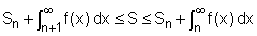For the given series Σ(1/n3) with n = 5, the inequlities become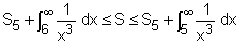The partial sum can be determined as follows: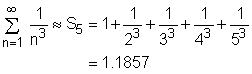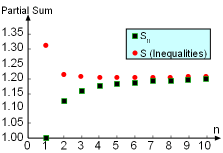The Partial Sum S Based on the Inequalities and Sn Subject to Different n Vales for the Series Σ(1/n3) The improper integral can be evaluated as: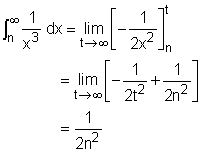The inequalities become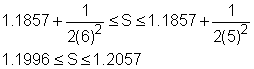Taking the midpoint as the total sum, hence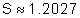with      Error = (1.2057 - 1.1996)/2 = 3.05 x 10-3